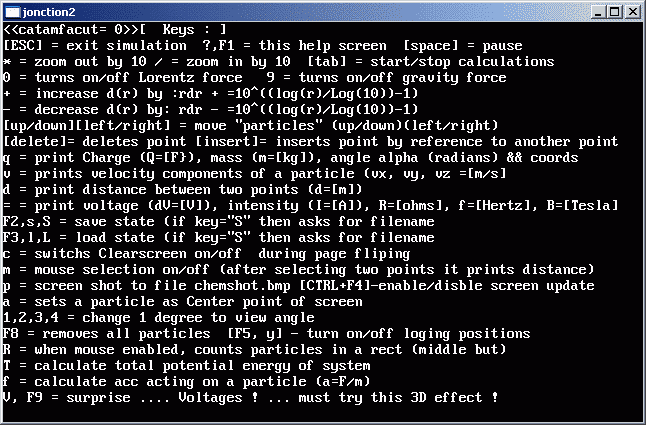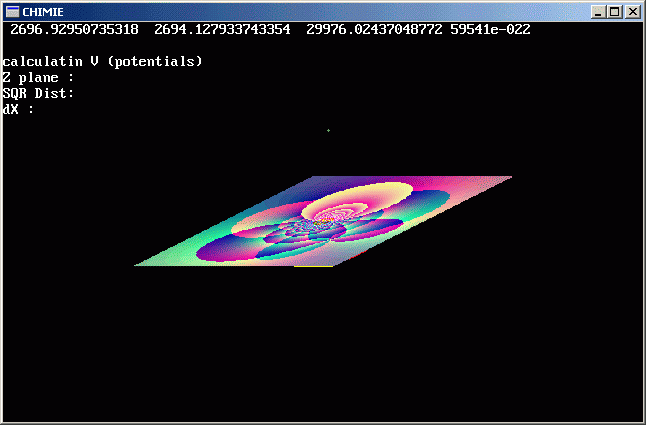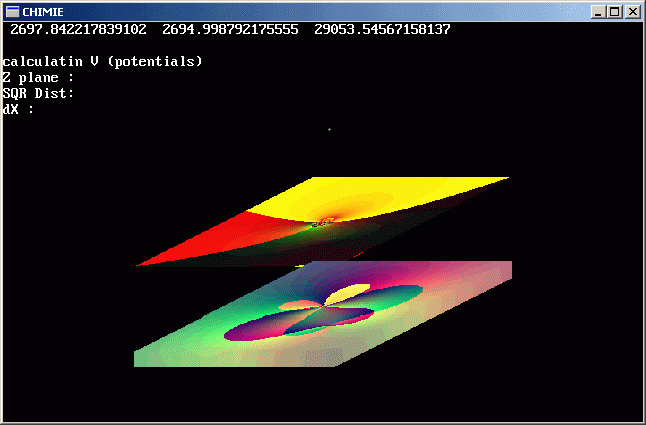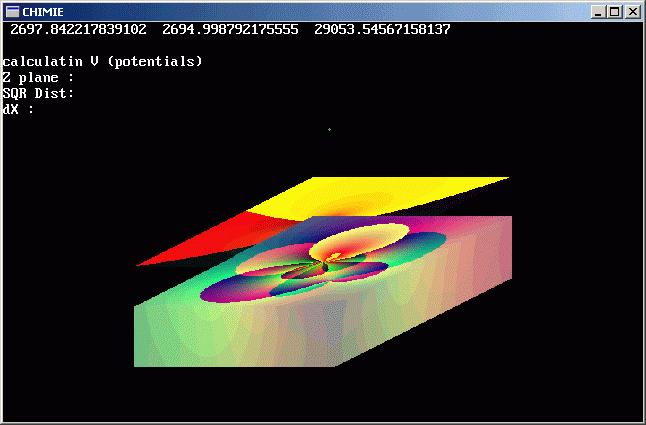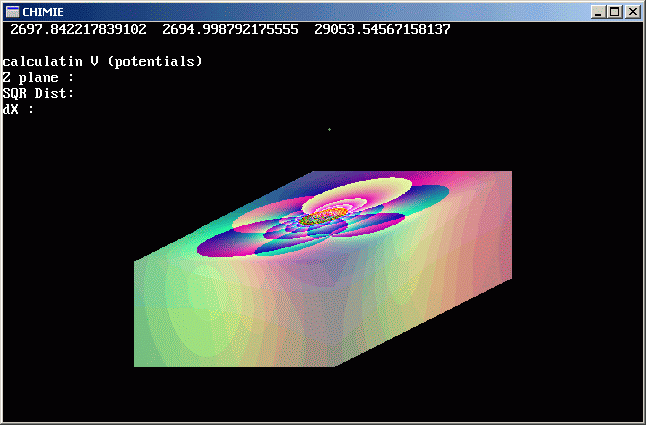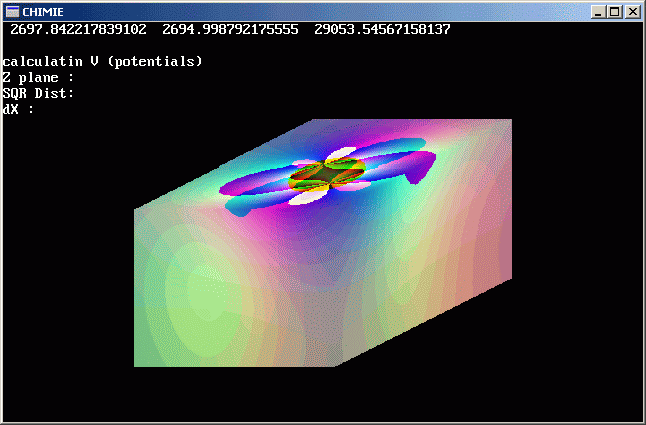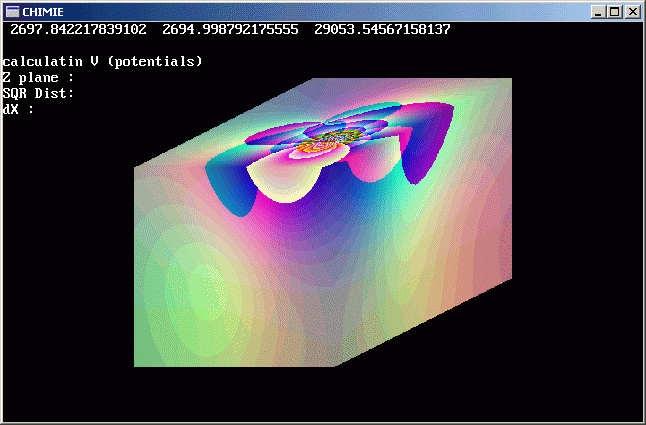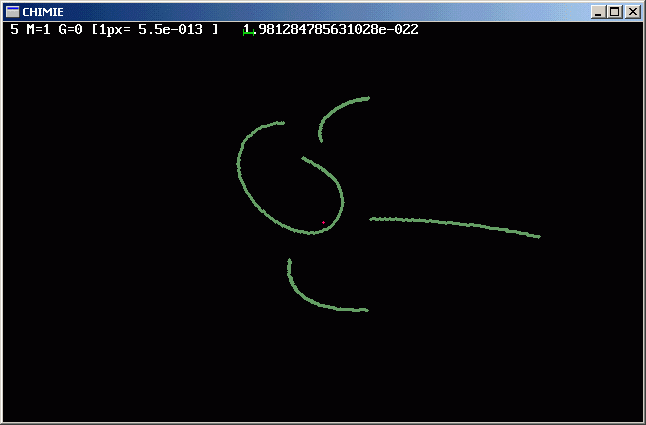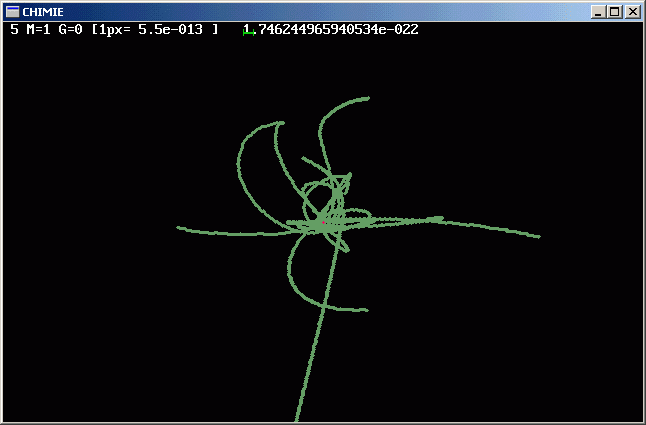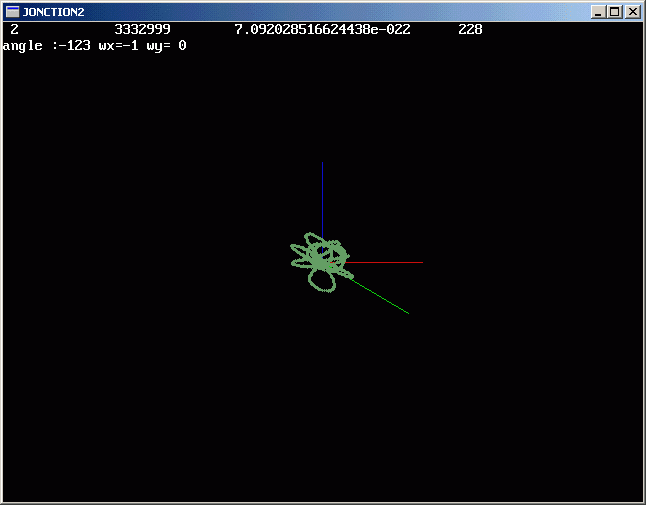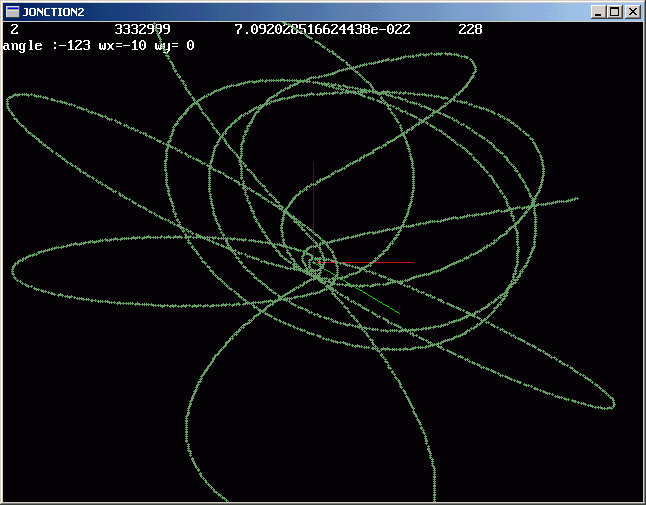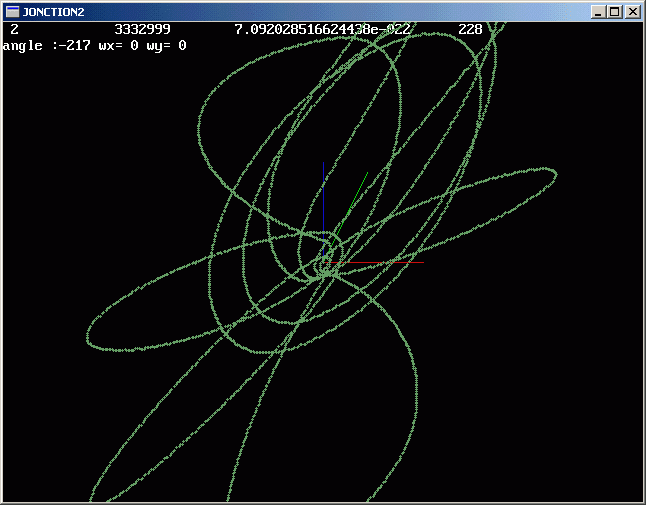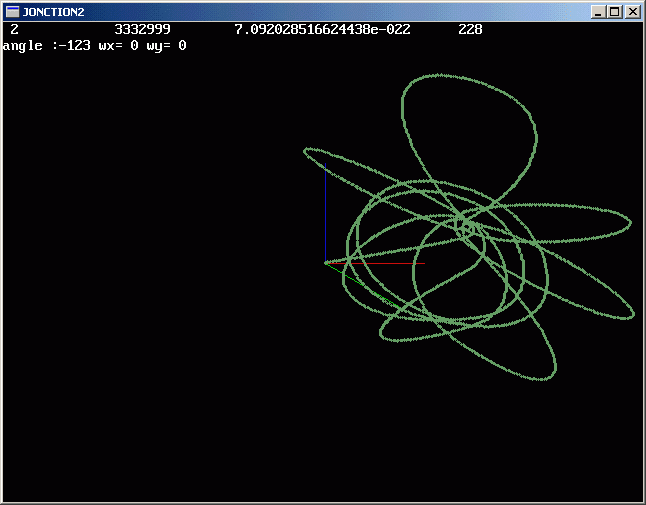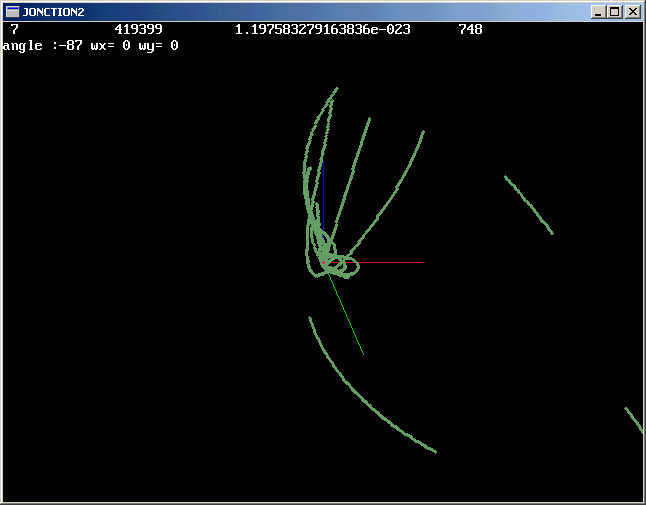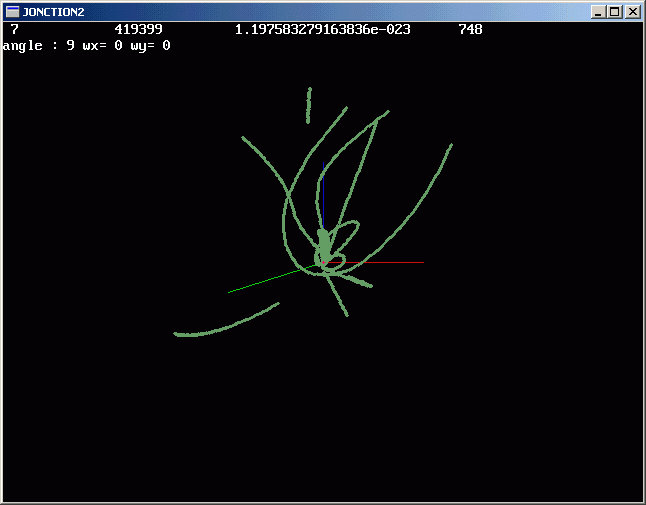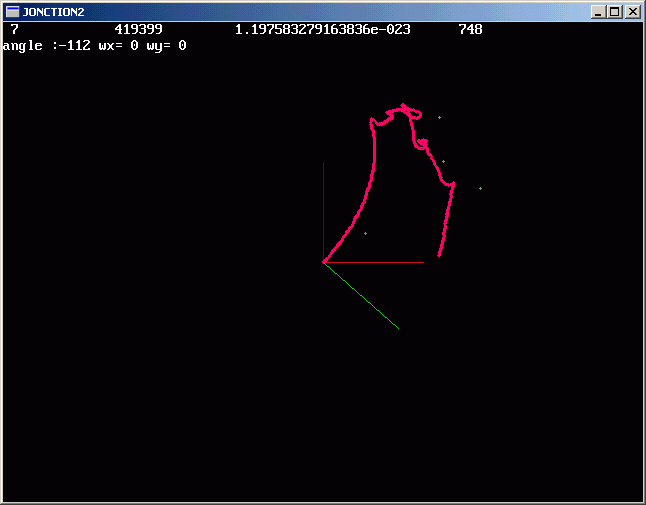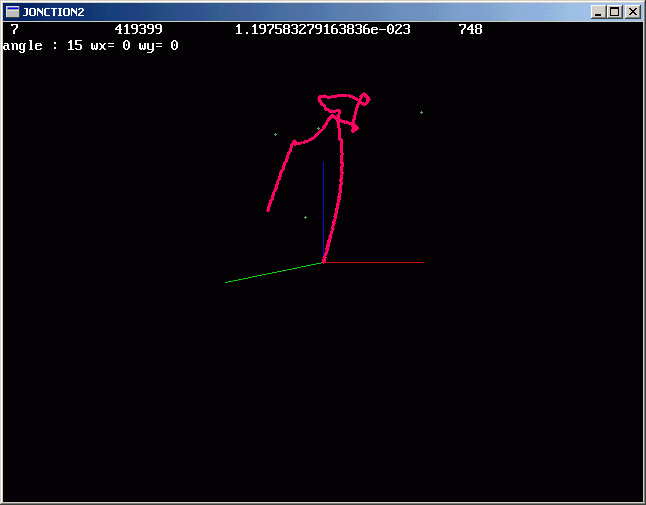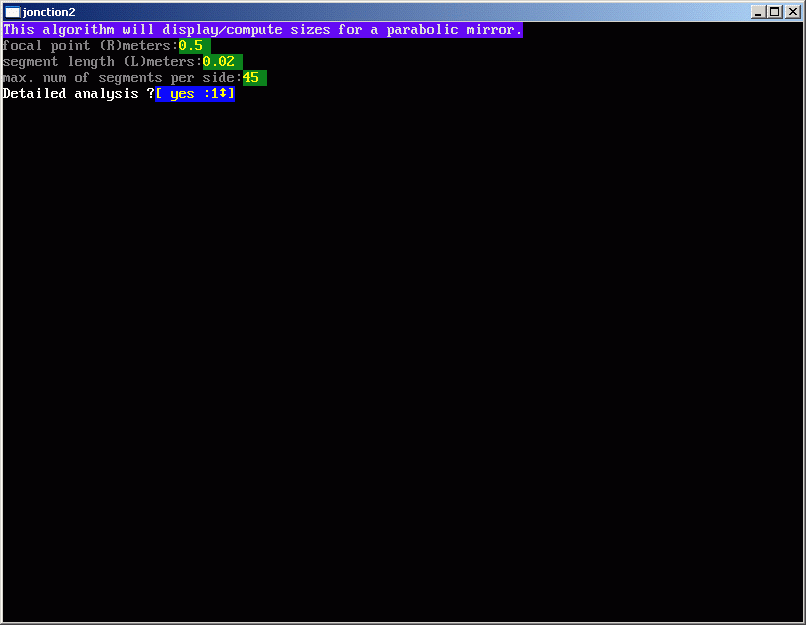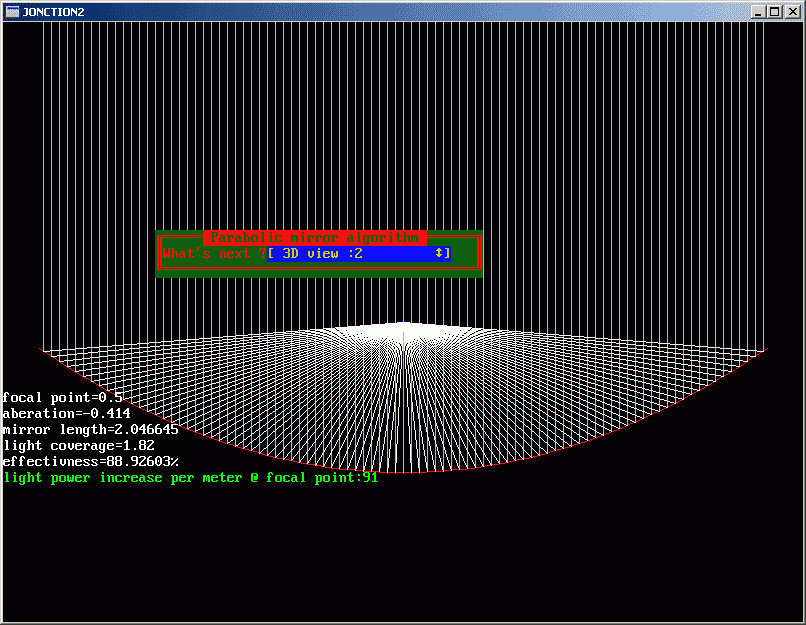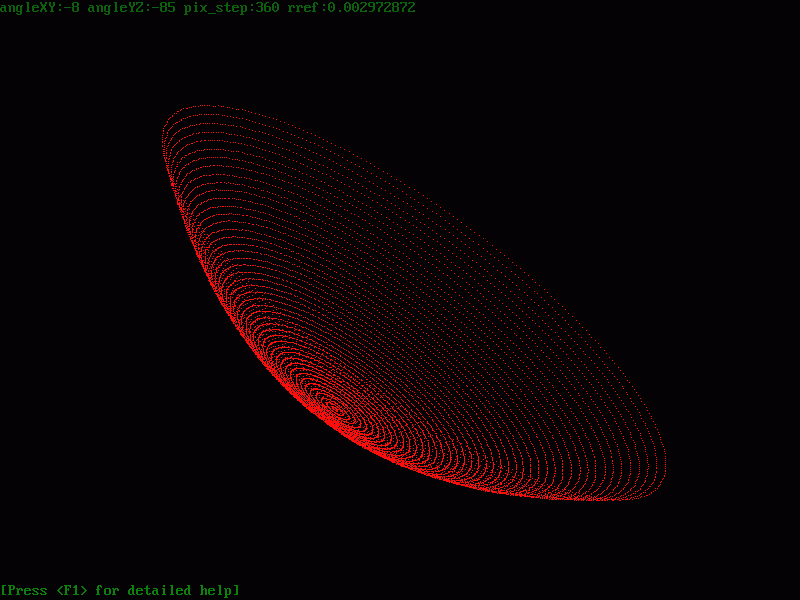DownloadJonction2 is now ver 2.01 ; it can: - for DIODES: compute (based on depletion approx.) and plot : xn,xp versus Nd; Id(Vab) versus Nd,Na,wp,wn; Id versus Vab,etc - for BJT : Ie(vbc,vbe), Ic(vbc,vbe), Ib .... - for MOS-capacitor : - for MOSFET: (using gradual channel approx.) Vt, Vfb, etc. - for SPUTTER: lambda vs p; E vs V; n vs I; J,Jsub, Vgrowth - for EVAPORATION: J vs (p, T); film growth rate; v growth vs time, temp, pressure, geometric factors, etc - for DIFFUSION: for surface source (exp), diffusion length for constat source (vapor source; erfc) - for CVD: film growth rate, etc. - for OXIDATION: compute oxide growth rate,etc -under implementation- - for CHEMICAL ETCH:....-under implementation- - for PHYSICAL ETCH:....-under implementation- - for CHARGES: electrodynamics+gravity (including Lorentz Force) real time simulation - for ELECTRO-MAGNETIC WAVES: power received at receiver, max. bandwidth,etc - for SIMIMULATION: the program has the ability to log data to disk and then display particle 3D "traces" for a variable amount of data. - for CHEMICAL-ELEMENTS: elements datasheets with constants (density, ionizing energy, atomic radius, etc) - for MIRRORS: parabolic mirror algorithm to compute geometrical values + 3D viewer of parabolic shape - compute spectral lines (momentanly using simple formula) - do heating analysis (compute energies need to heat materials) - compute parameters for storing energy using gravity and masses - forces transformations - do analysis for physical chemistry The main purpose of Jonction2 is to offer complete calculation of parameters needed to grow a double-jonction diode solar cell : Donor, Acceptor concetration(s); jonctions physical length; voltage, intensity, pressure and time needed to grow each jonction using sputtering. As an add-on, it contains the library [chimie.dll] . You can use this library to visualize PUNCTUAL CHARGE DYNAMICS. It computes, using superposition, total electric force, electric field, magnetic force, gravitational force acting on charged/uncharged SPHERES with mass as dimension. The minimal distance at which the electric, magnetic and gravitational forces are still acting on two particles/masses is set to be : E=m*c2 / (1-v2/c2) = q1 * q2 / (4 * π * r2) = G * m1 * m2 / r2 Also for Lorentz force:E=m*c2 / (1-v2/c2) = q1 * v x B x dr The actual used form in the program is more complex .... See source for details .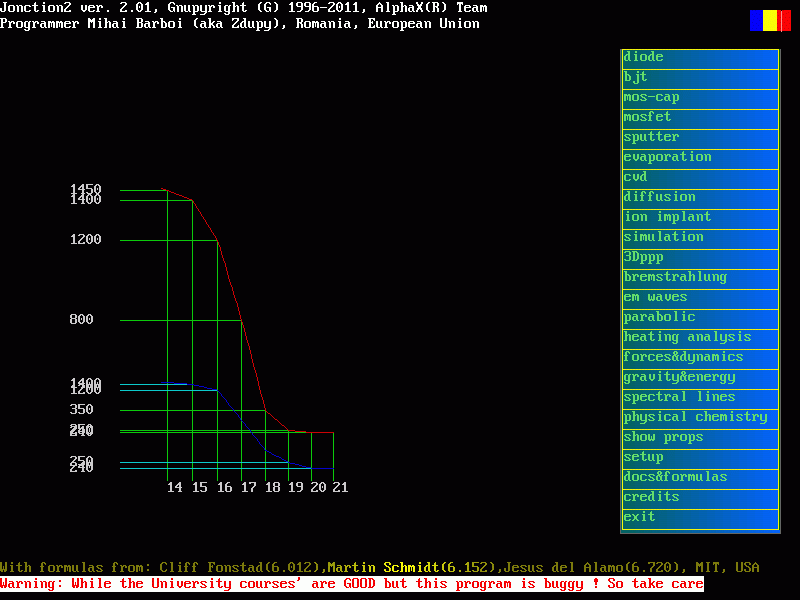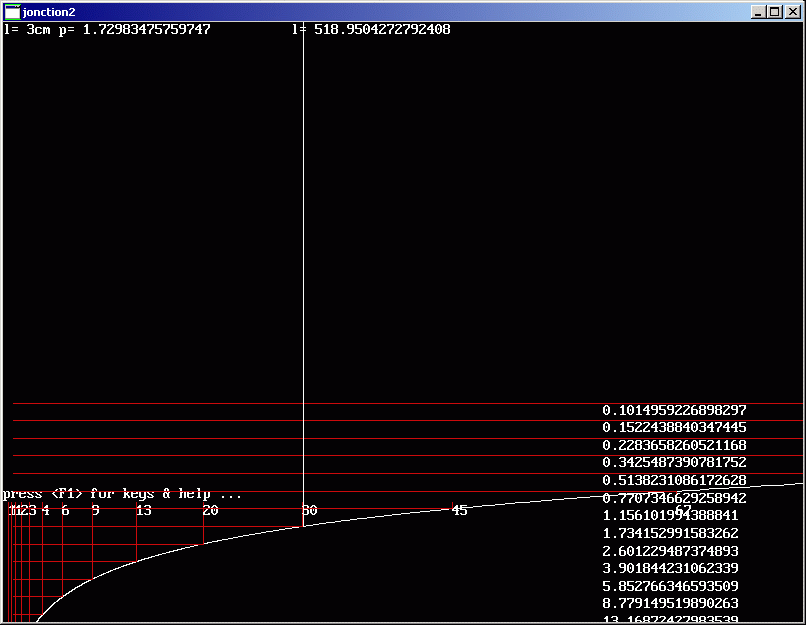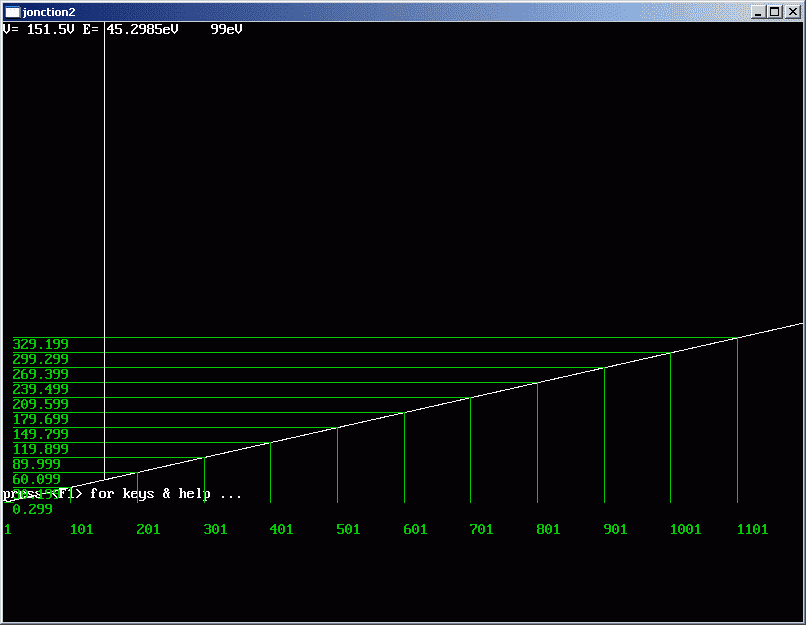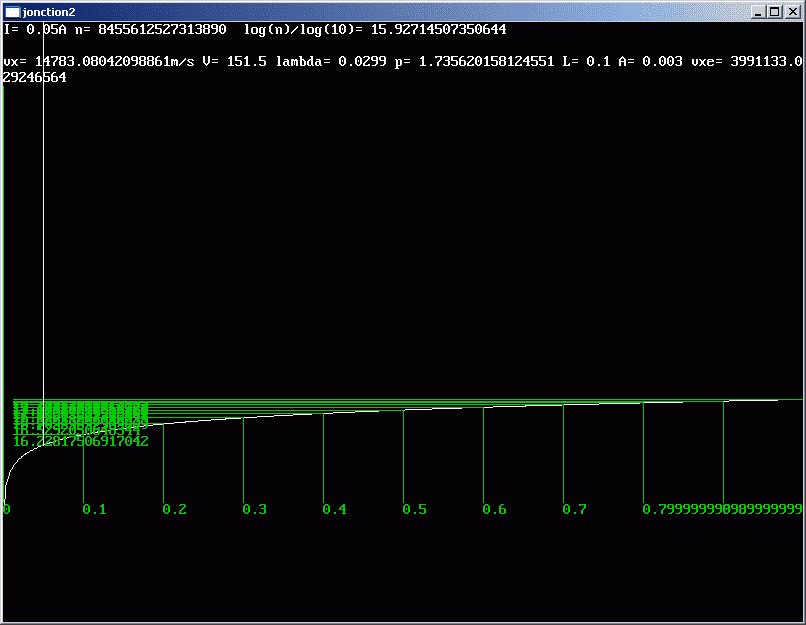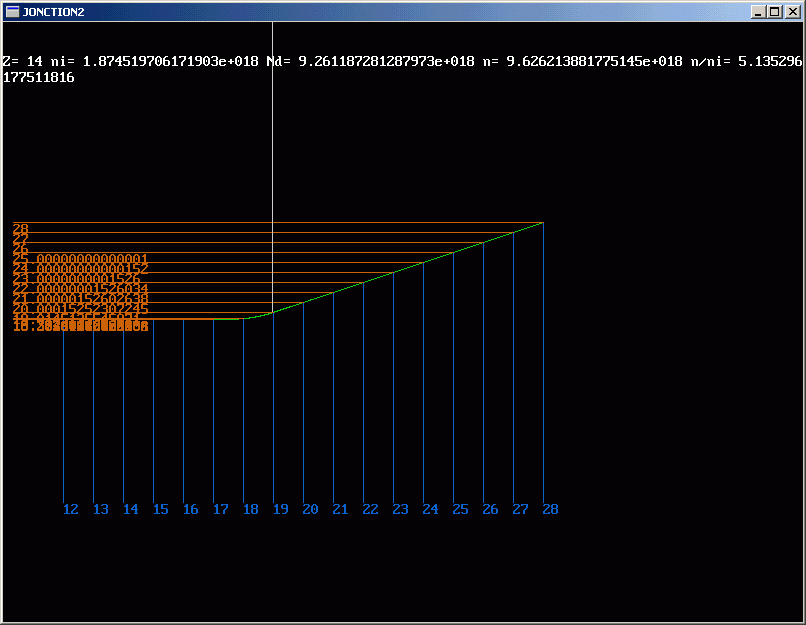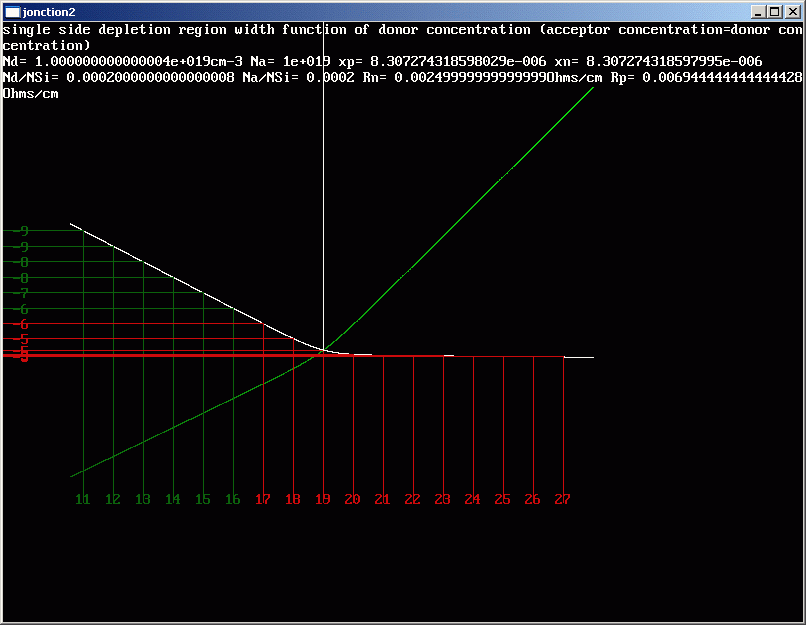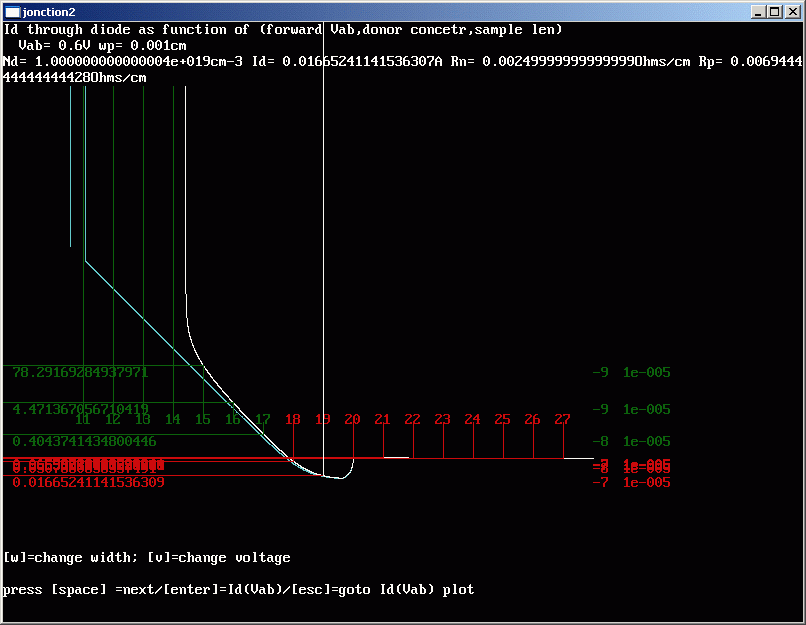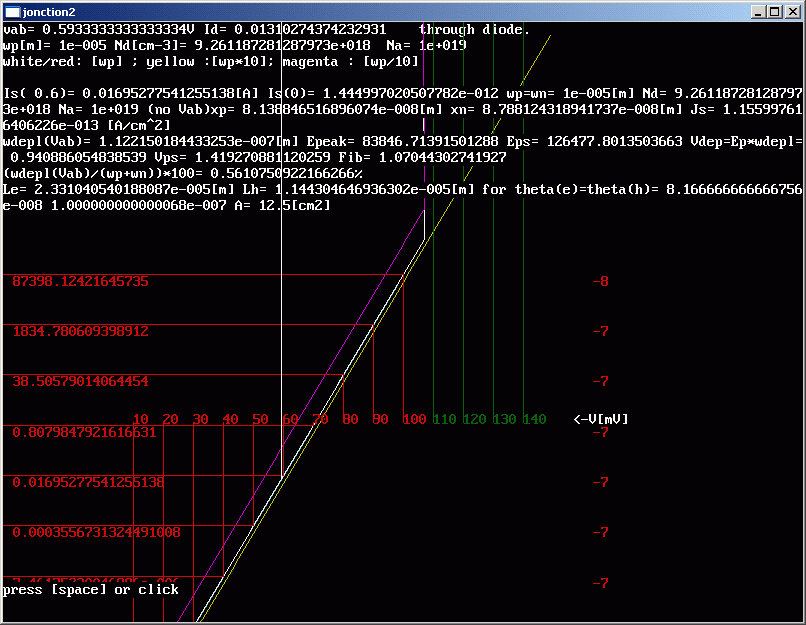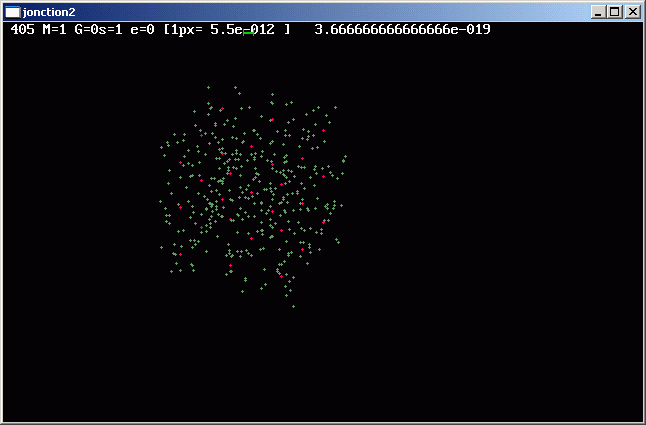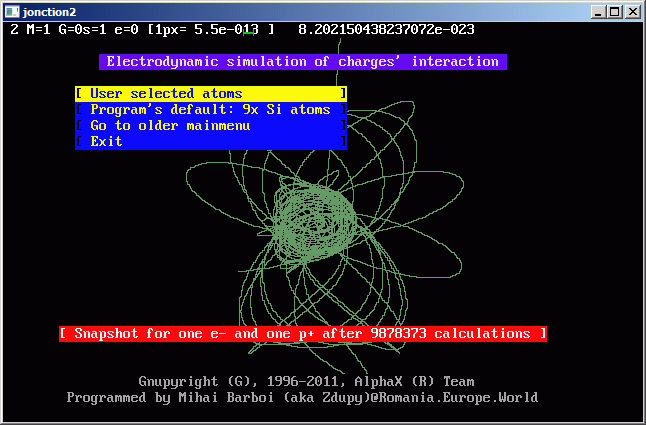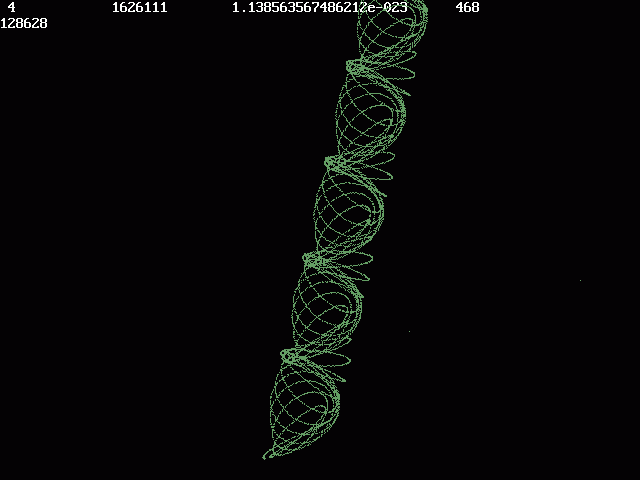chimie.dll is a part of Jonction2 now ....